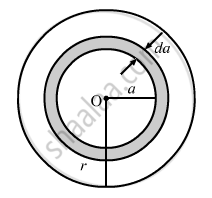Advertisement Remove all ads

# A Conducting Disc of Radius R Rotates with a Small but Constant Angular Velocity ω About Its Axis. a Uniform Magnetic Field B Exists Parallel to the Axis of Rotation. - Physics

Sum

A conducting disc of radius r rotates with a small but constant angular velocity ω about its axis. A uniform magnetic field B exists parallel to the axis of rotation. Find the motional emf between the centre and the periphery of the disc.

Advertisement Remove all ads

#### Solution

The angular velocity of the disc is ω. Also, the magnetic field of magnitude B is perpendicular to the disc.Let us take a circular element of thickness da at a distance a from the centre.

Linear speed of the element at a from the centre, v = ωa

Now,

$de = Blv$

$de = B \times da \times a\omega$

$\Rightarrow e = \int_0^r \left( B\omega a \right)da$

$e = \frac{1}{2}B\omega r^2$

Concept: Motional Electromotive Force
Is there an error in this question or solution?
Advertisement Remove all ads

#### APPEARS IN

HC Verma Class 11, Class 12 Concepts of Physics Vol. 2
Chapter 16 Electromagnetic Induction
Q 52 | Page 309
Advertisement Remove all ads

#### Video TutorialsVIEW ALL 

Advertisement Remove all ads
Share
Notifications

View all notifications

Forgot password?